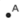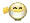OBJECTIVE: To learn how to identify the grid coordinates of points on the Cartesian plane

In a previous lesson, you were able to practice plotting points on the Cartesian plane using thePoint tool. You were given a set of ordered pairs, and you had to plot the points and connect them. In this exercise, the task is reversed. You are given a point (the location of the submarine), and you have to identify its coordinates by moving the x- and y-sliders. If you identify the coordinates correctly, you'll see ahappy face appear. Otherwise, you have to continue hunting for the sub. Record the coordinates of the submarine for each new location. Repeat the exercise until you have recorded at least one pair of grid coordinates for each of the four quadrants.

QUESTION: What were the farthest grid coordinates you were able to record in each of the four quadrants? (Answers vary.)

YOU'VE done practice on both plotting grid coordinates and reading grid coordinates.

Coordinates are useful in identifying the location of points in a plane. Next time, let's do geometric constructions involving the locus of a moving point, first in one direction and then in the opposite direction. You'll surely ENJOY it!